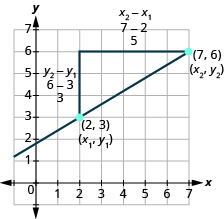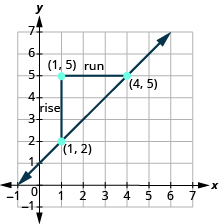## Finding Slope Given Two Points on a Line

### Learning Outcomes

• Find the slope of a line given two points on the line

Sometimes we need to find the slope of a line between two points and we might not have a graph to count out the rise and the run. We could plot the points on grid paper, then count out the rise and the run, but there is a way to find the slope without graphing.

Before we get to it, we need to introduce some new algebraic notation. We have seen that an ordered pair $\left(x,y\right)$ gives the coordinates of a point. But when we work with slopes, we use two points. How can the same symbol $\left(x,y\right)$ be used to represent two different points?

Mathematicians use subscripts to distinguish between the points. A subscript is a small number written to the right of, and a little lower than, a variable.

• $\left({x}_{1},{y}_{1}\right)\text{ read }x\text{ sub }1,y\text{ sub }1$
• $\left({x}_{2},{y}_{2}\right)\text{ read }x\text{ sub }2,y\text{ sub }2$

We will use $\left({x}_{1},{y}_{1}\right)$ to identify the first point and $\left({x}_{2},{y}_{2}\right)$ to identify the second point. If we had more than two points, we could use $\left({x}_{3},{y}_{3}\right),\left({x}_{4},{y}_{4}\right)$, and so on.

To see how the rise and run relate to the coordinates of the two points, let’s take another look at the slope of the line between the points $\left(2,3\right)$ and $\left(7,6\right)$ below.Since we have two points, we will use subscript notation.

$\stackrel{{x}_{1},{y}_{1}}{\left(2,3\right)}\stackrel{{x}_{2},{y}_{2}}{\left(7,6\right)}$

On the graph, we counted the rise of $3$. The rise can also be found by subtracting the $y\text{-coordinates}$ of the points.

$\begin{array}{c}{y}_{2}-{y}_{1}\\ 6 - 3\\ 3\end{array}$

We counted a run of $5$. The run can also be found by subtracting the $x\text{-coordinates}$.

$\begin{array}{c}{x}_{2}-{x}_{1}\\ 7 - 2\\ 5\end{array}$

 We know $m={\Large\frac{\text{rise}}{\text{run}}}$ So $m={\Large\frac{3}{5}}$ We rewrite the rise and run by putting in the coordinates. $m={\Large\frac{6 - 3}{7 - 2}}$ But $6$ is the $y$ -coordinate of the second point, ${y}_{2}$ and $3$ is the $y$ -coordinate of the first point ${y}_{1}$ . So we can rewrite the rise using subscript notation. $m={\Large\frac{{y}_{2}-{y}_{1}}{7 - 2}}$ Also $7$ is the $x$ -coordinate of the second point, ${x}_{2}$ and $2$ is the $x$ -coordinate of the first point ${x}_{2}$ . So we rewrite the run using subscript notation. $m={\Large\frac{{y}_{2}-{y}_{1}}{{x}_{2}-{x}_{1}}}$

We’ve shown that $m={\Large\frac{{y}_{2}-{y}_{1}}{{x}_{2}-{x}_{1}}}$ is really another version of $m={\Large\frac{\text{rise}}{\text{run}}}$. We can use this formula to find the slope of a line when we have two points on the line.

### Slope Formula

The slope of the line between two points $\left({x}_{1},{y}_{1}\right)$ and $\left({x}_{2},{y}_{2}\right)$ is

$m={\Large\frac{{y}_{2}-{y}_{1}}{{x}_{2}-{x}_{1}}}$

$\text{Slope is }y\text{ of the second point minus }y\text{ of the first point}$
$\text{over}$
$x\text{ of the second point minus }x\text{ of the first point.}$

Doing the Manipulative Mathematics activity “Slope of Lines Between Two Points” will help you develop a better understanding of how to find the slope of a line between two points.

### example

Find the slope of the line between the points $\left(1,2\right)$ and $\left(4,5\right)$.

Solution

 We’ll call $\left(1,2\right)$ point #1 and $\left(4,5\right)$ point #2. $\stackrel{{x}_{1},{y}_{1}}{\left(1,2\right)}\text{and}\stackrel{{x}_{2},{y}_{2}}{\left(4,5\right)}$ Use the slope formula. $m={\Large\frac{{y}_{2}-{y}_{1}}{{x}_{2}-{x}_{1}}}$ Substitute the values in the slope formula: $y$ of the second point minus $y$ of the first point $m={\Large\frac{5 - 2}{{x}_{2}-{x}_{1}}}$ $x$ of the second point minus $x$ of the first point $m={\Large\frac{5 - 2}{4 - 1}}$ Simplify the numerator and the denominator. $m={\Large\frac{3}{3}}$ $m=1$

Let’s confirm this by counting out the slope on the graph.The rise is $3$ and the run is $3$, so
$\begin{array}{}\\ m=\frac{\text{rise}}{\text{run}}\hfill \\ m={\Large\frac{3}{3}}\hfill \\ m=1\hfill \end{array}$

### try it

How do we know which point to call #1 and which to call #2? Let’s find the slope again, this time switching the names of the points to see what happens. Since we will now be counting the run from right to left, it will be negative.

 We’ll call $\left(4,5\right)$ point #1 and $\left(1,2\right)$ point #2. $\stackrel{{x}_{1},{y}_{1}}{\left(4,5\right)}\text{and}\stackrel{{x}_{2},{y}_{2}}{\left(1,2\right)}$ Use the slope formula. $m={\Large\frac{{y}_{2}-{y}_{1}}{{x}_{2}-{x}_{1}}}$ Substitute the values in the slope formula: $y$ of the second point minus $y$ of the first point $m={\Large\frac{2 - 5}{{x}_{2}-{x}_{1}}}$ $x$ of the second point minus $x$ of the first point $m={\Large\frac{2 - 5}{1 - 4}}$ Simplify the numerator and the denominator. $m={\Large\frac{-3}{-3}}$ $m=1$

The slope is the same no matter which order we use the points.

### example

Find the slope of the line through the points $\left(-2,-3\right)$ and $\left(-7,4\right)$.

### try it

Watch this video to see more examples of how to determine slope given two points on a line.